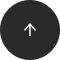SQL Server

# How to Get Yesterday’s Date in T-SQL

#### Problem:

You would like to display yesterday's date (without time) in an SQL Server database.

#### Solution:

```SELECT DATEADD(day, -1, CAST(GETDATE() AS date)) AS YesterdayDate;
```

Assuming today is 2020-09-24, the result is:

yesterday_date
2020-09-23

#### Discussion:

To get yesterday's date, you need to subtract one day from today's date. Use `GETDATE()` to get today's date (the type is `datetime`) and cast it to `date`. In SQL Server, you can subtract or add any number of days using the `DATEADD()` function.

The `DATEADD()` function takes three arguments: `datepart`, `number`, and `date`. Here, the value of `datepart` is `day`, because the unit of time you want to subtract is day. The second argument is -1 (you subtract 1 day, which is the same as adding -1 day). The third argument is today's date—the date from which you want to subtract.

Of course, you can go back by any interval of time just as easily. Here's an example:

```SELECT DATEADD(month, -5, CAST(GETDATE() AS date));
```

An interval of time can also be `added` to a date. So, here’s a way if you want to get tomorrow's date:

```SELECT DATEADD(day, 1, CAST(GETDATE() AS date)) AS TomorrowDate;
```

#### Recommended articles: